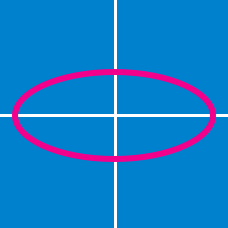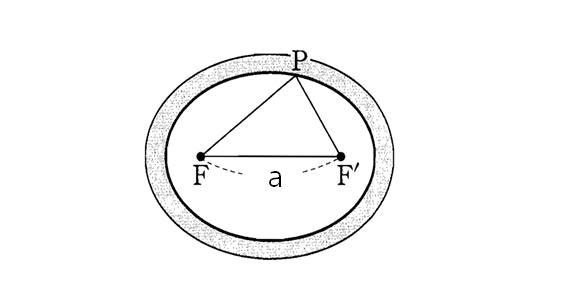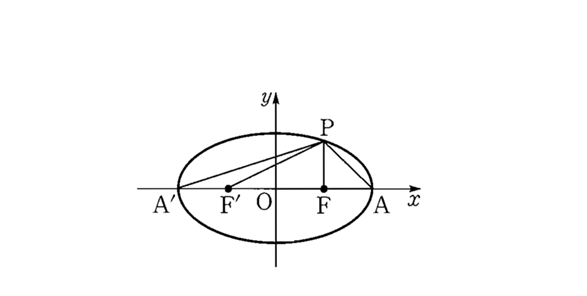Geometry

# Conics - Ellipse - Foci

Suppose $F$ and $F'$ are the foci of the ellipse $\displaystyle{\frac{x^2}{121}+\frac{y^2}{64}=1}.$ If $P$ is a point on the ellipse and $\lvert \overline{FP} \rvert$ denotes the length of $\overline{FP},$ what the minimum value of $\lvert \overline{FP} \rvert^2 + \lvert \overline{F'P} \rvert^2?$The above diagram is an ellipse-shaped track. The distance between the two foci $F$ and $F'$ is $8,$ and the sum of the distances between any point $P$ on the inner side of the track and $F$ and $F'$ is $32.$ What is the maximum area of $\triangle PF'F?$

For an ellipse $E$ with foci $A=(2,0)$ and $B=(10,0),$ every point $P$ on $E$ satisfies $\lvert PA \rvert + \lvert PB \rvert = 30.$ What is the equation of ellipse $E?$

Note: $\lvert PA \rvert$ denotes the length of line segment $PA$.In the above diagram, $F$ and $F'$ are the foci of the ellipse $\frac{x^2}{a^2}+\frac{y^2}{b^2}=1$ and $A$ and $A'$ are the end points of the major axis of the ellipse. If the area of triangle $\triangle A'PA$ is double the area of triangle $\triangle F'PF$ and the perimeter of triangle $\triangle F'PF$ is $66,$ what is the value of $a^2+b^2?$

What is the equation of the circle centered at $(3,0)$ which passes through the foci of the ellipse $\frac{x^{2}}{9} + \frac{y^{2}}{16} = 1?$

×